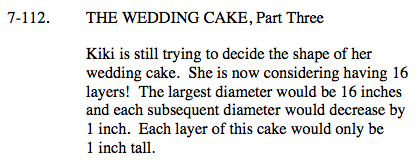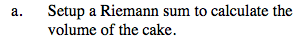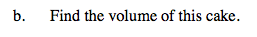### Home > CALC > Chapter Ch7 > Lesson 7.3.1 > Problem7-112

7-112.
1. THE WEDDING CAKE, Part Three

2. Kiki is still trying to decide the shape of her wedding cake. She is now considering having 16 layers! The largest diameter would be 16 inches and each subsequent diameter would decrease by 1 inch. Each layer of this cake would only be 1 inch tall. Homework Help ✎

1. Setup a Riemann sum to calculate the volume of the cake.

2. Find the volume of this cake.Since there are 16 layers of cake, and 16 is a large number, it would be beneficial to write the Riemann sum in sigma notation:

Δx represents the height of each cylindrical layer of cake.

Figure out the radius of the smallest layer. This will give you the initial value a.No need to expand. Use a calculator to evaluate the sigma notation.